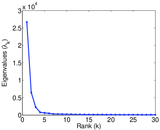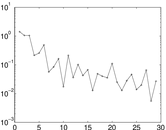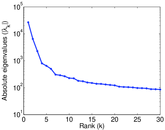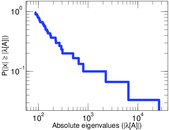# Wiktionary edits (hy)

This is the bipartite edit network of the Armenian Wiktionary. It contains users and pages from the Armenian Wiktionary, connected by edit events. Each edge represents an edit. The dataset includes the timestamp of each edit.

 Code `mhy` Internal name `edit-hywiktionary` Name Wiktionary edits (hy) Data source http://dumps.wikimedia.org/ AvailabilityDataset is available for download Consistency checkDataset passed all tests Category Authorship network Dataset timestamp 2017-10-20 Node meaning User, article Edge meaning Edit Network formatBipartite, undirected Edge typeUnweighted, multiple edges Temporal dataEdges are annotated with timestamps

## Statistics

 Size n = 218,585 Left size n1 = 1,202 Right size n2 = 217,383 Volume m = 1,183,865 Unique edge count m̿ = 634,076 Wedge count s = 20,541,171,816 Claw count z = 918,766,533,443,506 Cross count x = 3.330 75 × 1019 Square count q = 8,718,756,758 4-Tour count T4 = 151,916,021,340 Maximum degree dmax = 438,603 Maximum left degree d1max = 438,603 Maximum right degree d2max = 26,672 Average degree d = 10.832 1 Average left degree d1 = 984.913 Average right degree d2 = 5.445 99 Fill p = 0.002 426 67 Average edge multiplicity m̃ = 1.867 07 Size of LCC N = 218,165 Diameter δ = 14 50-Percentile effective diameter δ0.5 = 1.998 96 90-Percentile effective diameter δ0.9 = 3.872 71 Median distance δM = 2 Mean distance δm = 3.051 90 Gini coefficient G = 0.716 592 Balanced inequality ratio P = 0.234 987 Left balanced inequality ratio P1 = 0.064 305 5 Right balanced inequality ratio P2 = 0.349 817 Power law exponent γ = 2.104 79 Tail power law exponent γt = 1.521 00 Tail power law exponent with p γ3 = 1.521 00 p-value p = 0.065 000 0 Left tail power law exponent with p γ3,1 = 1.521 00 Left p-value p1 = 0.046 000 0 Right tail power law exponent with p γ3,2 = 4.451 00 Right p-value p2 = 0.000 00 Degree assortativity ρ = −0.100 527 Degree assortativity p-value pρ = 0.000 00 Spectral norm α = 26,692.2 Algebraic connectivity a = 0.007 170 95 Spectral separation |λ1[A] / λ2[A]| = 4.121 78 Controllability C = 216,152 Relative controllability Cr = 0.989 480

## Plots

### Fruchterman–Reingold graph drawing### Degree distribution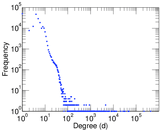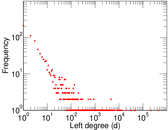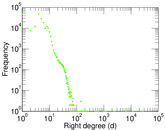### Cumulative degree distribution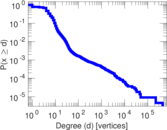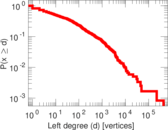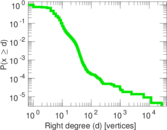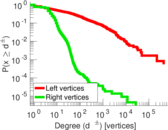### Lorenz curve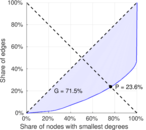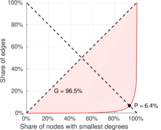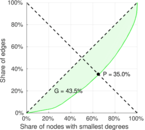### Spectral distribution of the adjacency matrix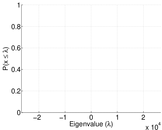### Spectral distribution of the normalized adjacency matrix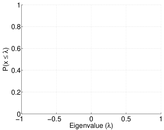### Spectral distribution of the Laplacian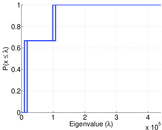### Spectral graph drawing based on the adjacency matrix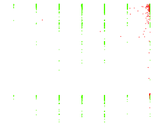### Spectral graph drawing based on the Laplacian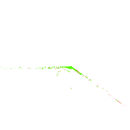### Spectral graph drawing based on the normalized adjacency matrix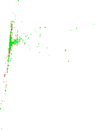### Degree assortativity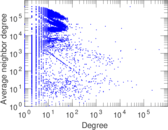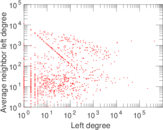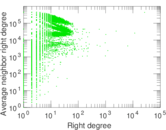### Zipf plot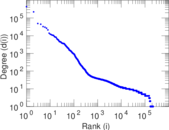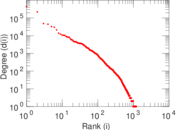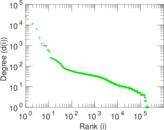### Hop distribution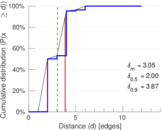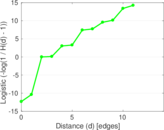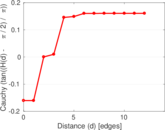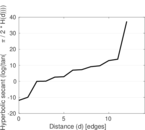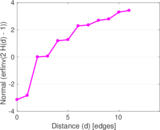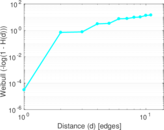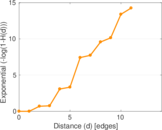### Delaunay graph drawing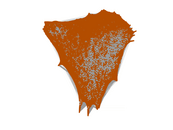### Edge weight/multiplicity distribution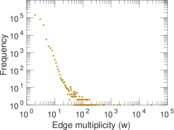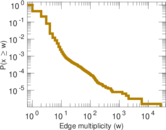### Temporal distribution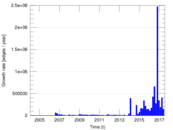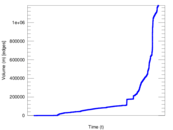### Diameter/density evolution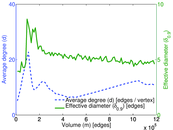### Matrix decompositions plots